# Designing and Controlling Quadruped Spider Robot with ESP32

Published  August 25, 2023   0
G
Author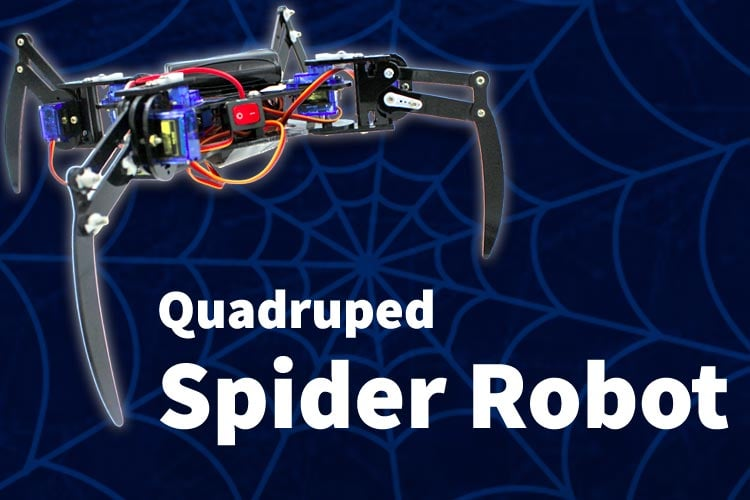Nature has always been an incredible source of inspiration for technological advancements and engineers have sought to replicate the intricate mechanisms found in various animals. One such remarkable creation is the spider robot. It can also known as a quadruped robot due to its four legs. This innovative robotic project aims to emulate the locomotion and behavior of spiders. Learn more using our previously built robotic projects.

Spider robot is a four-legged walking robot which is inspired by or a bionic replica of spider that uses their legs for movement. The legs are arranged in a way that allows them to move in a variety of ways, including walking, running, and climbing. An integral component of this project is the integration of the ESP32 microcontroller, which enables wireless control and advanced motion coordination. The ESP32 serves as an ideal platform for the spider robot, enhancing its agility, versatility, and capacity to navigate complex terrains effectively. With their exceptional agility, versatility, and ability to traverse complex terrains, spider robots hold immense potential in various fields, including search and rescue missions, exploration, and even household assistance.

### Mechanism of Spider Robot

The mechanism of a spider robot involves several key components and principles that enable its locomotion and functionality.

Spider robots belong to the category of legged robots, which are typically more intricate than wheeled robots. Legged robots excel in traversing challenging terrains compared to wheeled robots. While wheeled robots usually have DC motors whereas legged robots generally have servo motors.

One fundamental distinction between wheeled and legged robots lies in their degrees of freedom (DOF). Wheeled robots only have one degree of freedom whereas a legged robot has a more complex motion.

Degrees of freedom (DOF) of a body are defined by the number of parameters it takes to define the position of that same body. In the case of our spider robot have 2 servo motors in each of its legs to give out 2 different directions of motion. This means that the degree of freedom (DOF) for one leg is 2. So the total degrees of freedom (DOF) of spider robot is 8.

This project might not be very complex in terms of the wiring and the assembly, but it is incredibly complex to understand the kinematics behind the motion of the quadruped spider robot.

To achieving controlled movement, the robot has to perform a different number of poses that collectively generate a single motion. It can be done by implementing crawl gait (pattern of movement) in which one leg will be in air and remaining three legs should be in contact with the ground while moving. Typically, to take one step forward, the robot undergoes 6 distinct poses.

#### Quadruped Gait for Forward Movement

Gait refers to the specific pattern of leg movement required to propel the body towards a desired destination. In the case of quadruped robots, the gait involves lifting one leg at a time while the remaining three legs form a supportive tripod. This coordinated movement ensures stability and efficient locomotion.

To achieve quadruped gait, the key is to determine when and at what position each leg should move. This process is known as inverse kinematics. Inverse kinematics enables us to calculate the necessary values for the servo motors controlling the legs, allowing precise positioning of the legs to achieve the desired gait pattern.

Inverse kinematics plays a crucial role in the smooth execution of the quadruped gait. By providing the necessary instructions to the servo motors, the robot's legs can be accurately moved to their intended positions. This helps in maintaining balance and achieving efficient locomotion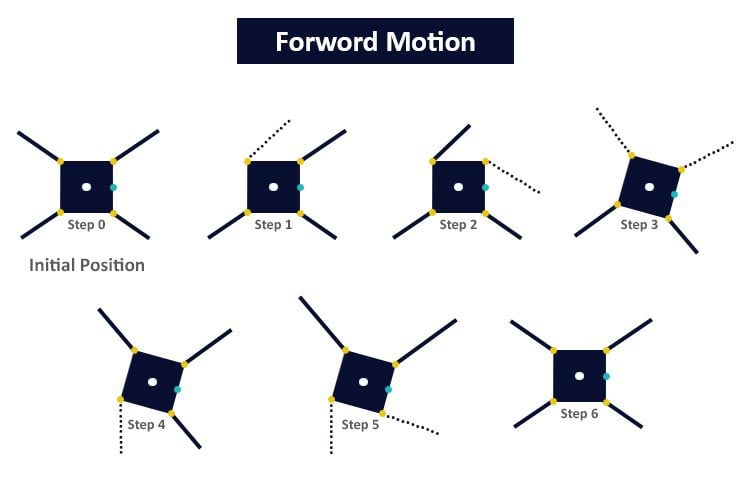The above diagram illustrates the forward quadruped gait, showcasing the sequence of leg movements required for the robot to move forward effectively. The dotted line shows that the foot has been lifted.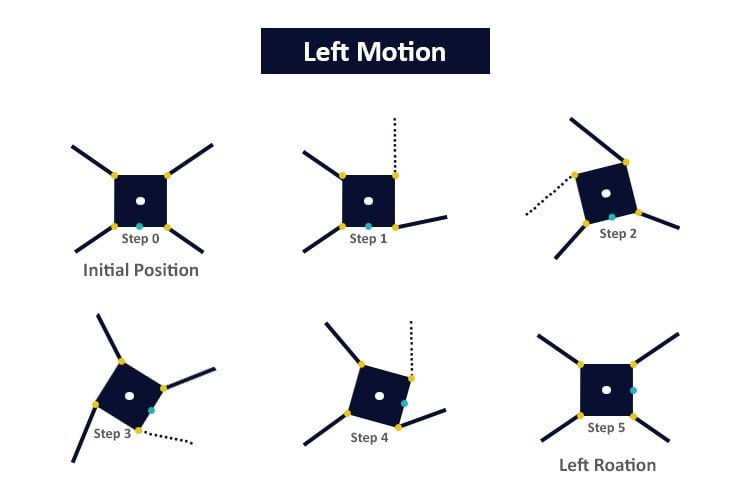To ensure the stability of the robot, it is crucial for the center of mass (COM) to remain within the support polygon. The support polygon refers to an imaginary polygon within which, if the COM is located, the robot can maintain its balance. However, if the COM shifts outside of the support polygon, the robot becomes unstable and may tip over. The concept is depicted in the figure below, where the robot remains balanced as long as the COM resides inside the support polygon. It serves as a critical principle for maintaining stability and preventing falls in the robot's operation.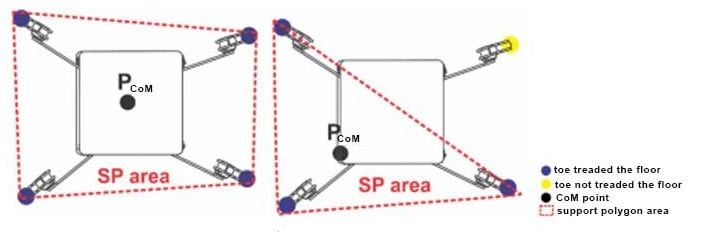### Components Required for Spider Robot

• ESP32 microcontroller
• SG90 plastic gear servo motor - 8pcs
• LM2596 DC-DC Buck converter
• 12V li-ion battery
• Switch
• Jumper wires
• Breadboard or PCB (Printed Circuit Board)
• Screws, nuts, spacers

### Spider Robot Laser Cutting Files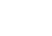Auadruped Spider robot Acrylic Body

We have designed the Quadruped Spider Robot Acrylic parts using SolidWorks. The .dxf files, you can find on the above link. If you want to make any changes, you can also find .svg file. These pieces are also readily available on many E-commerce websites, just search "Quadruped Spider Robot parts" on google and you will find many links.

### Assembly of Spider Robot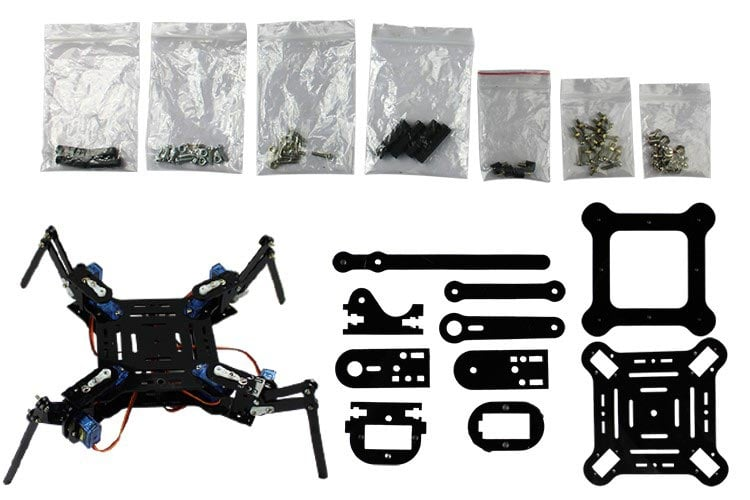Before starting the assembly, we need to adjust the angle adjustment of the servo motors. Otherwise, your robot will not work properly.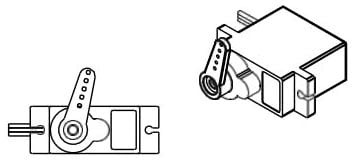To begin, attach the Servo Arm to the servo by carefully aligning and securing it in place. We can manually calibrate and adjust the servo motor angle but some time manually calibration is not accurate or may not be possible. So you can calibrate your servo motors using the provided code #1. Refer to the circuit diagram mentioned below and connect your servo motors to pins 21, 19, 33, 25, 27, 14, 12, and 13 accordingly. Upload the provided code #1 to your ESP32, which will facilitate the calibration process. Failing to execute this step may result in unstable performance of your robot.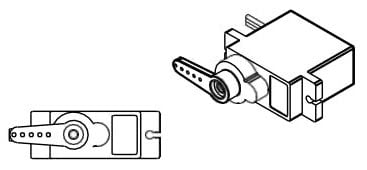By ensuring proper alignment and calibration of the servos, you lay the foundation for a stable and well-functioning robot.

After done with servo adjustments, you can pass to Leg Assembly stages.

### Leg Assembly Stages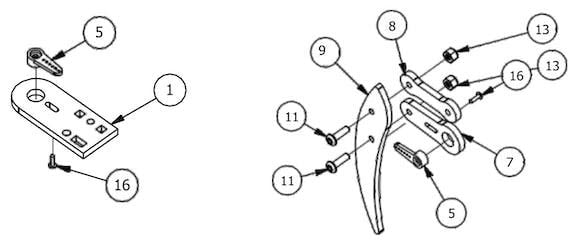Step 1: Take a servo single arm and place it on the pedestal pivot plate. Insert the servo mounting screw into the servo single arm from the rear side of the leg-up pivot plate. Tighten the screw until the servo single arm is securely attached to the pivot plate.

Step 2: Now, attach another servo single arm to the standing servo arm. To do this, insert a servo mounting screw from the back of the leg servo arm and fasten it into the servo single arm. Make sure the connection is firm and stable.

Step 3: Moving on, connect the leg parallel joint to the leg piece using an M3x10mm screw and an M3 fiber nut. Double-check the tightness of the screw to ensure the joint is firmly joined to the leg piece.

Step 4: Next, attach the leg piece to the leg servo arm using an M3x10mm screw and an M3 fiber nut.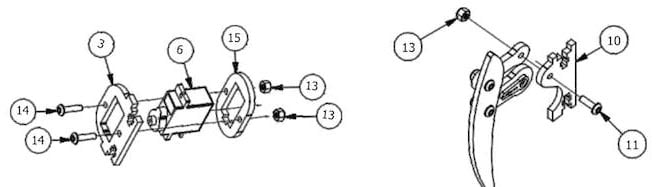Step 5: Insert another servo into the servo holder.

Step 6: Place the top end of the second servo into the pedestal servo slot.

Step 7: Fasten the servo holder to the leg servo socket using two M3x12mm screws and two M3 fiber nuts.

Step 8: Now, connect a leg parallel plate to the other end of the leg using an M3x10mm screw and an M3 fiber nut.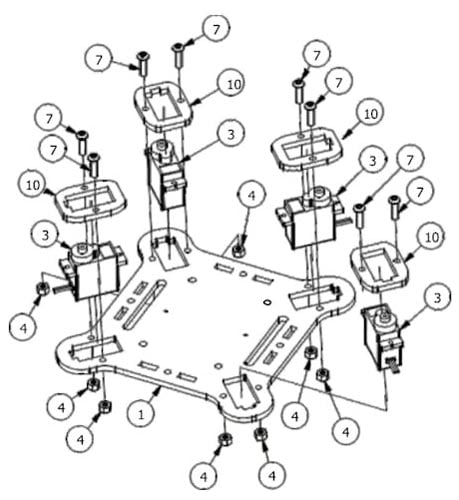Step 9: With both servos in the center position and the leg parallel joint horizontally aligned, attach the leg servo arm to the first servo using the supplied servo arm screw.

Step 10: Finally, join the Leg Assembly, which was completed in the previous steps, to the Leg Bottom Pivot Plate using two M3x10mm screws and two M3 nuts.

The Leg Assembly stage may have been challenging, but once these steps are completed, the remaining process becomes more manageable. If you have successfully finished these stages, let's proceed to the Body Assembly part.

### Body Assembly

In this stage, you should be careful with the numbers. Schematics is explained according to the part numbers, so that you should read the lists that we created, before the assembly.

Part List of Schema 1 & 2:

• 1- Body Upper Plate
• 2- M3x10MM Screw
• 3 - Servo Motor
• 4 - M3 Nut
• 5 - M3 Fiber Nut
• 7 - M3x12MM Screw
• 8 - Spacer
• 10 - Servo Holder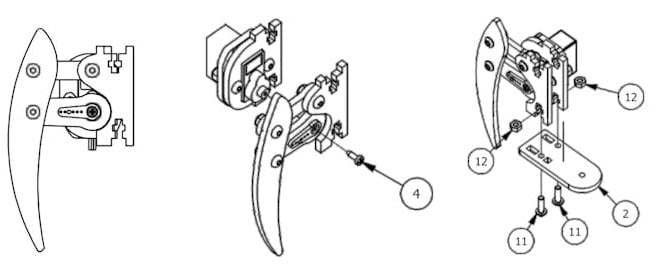Step 1: Begin the assembly by attaching the four stem spacers to the stem bottom plate. Use four M3x10mm screws and four M3 flat nuts to secure the spacers firmly in place.

Step 2: Take the four servos and position them on top of the chassis top plate, making sure they are correctly aligned.

Step 3: For each servo, attach a servo holder on top. Align the holder properly and secure it in place.

Step 4: Fasten each servo holder to the chassis top plate using M3x12mm screws and M3 fiber nuts. Ensure they are tightened securely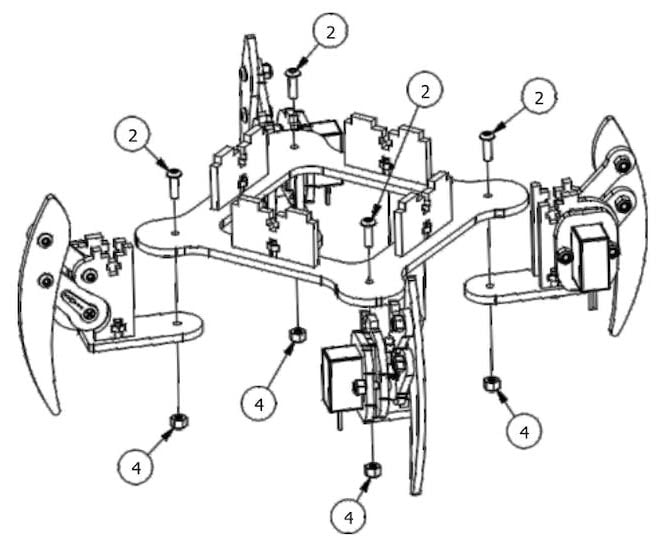Step 5: Now, proceed to attach each leg piece to the stem bottom plate. Use an M3x10mm screw and an M3 fiber nut for each leg piece. Be cautious not to over-tighten the screws, as it may lead to servo malfunctions.

Step 6: Bring together the body bottom plate and the body top plate. Use four M3x10mm screws and M3 nuts to firmly connect these two components.

Step 7: With the servos and leg pieces in place, carefully rotate each leg to a 45-degree angle as shown in the instructions. Install the leg upper pivot plate and secure it to each pivot servo and leg using two M3x10mm screws and two M3 nuts for each leg.

Step 8: Finally, secure the servo single arm to its respective servo using a servo screw, making sure it is firmly attached..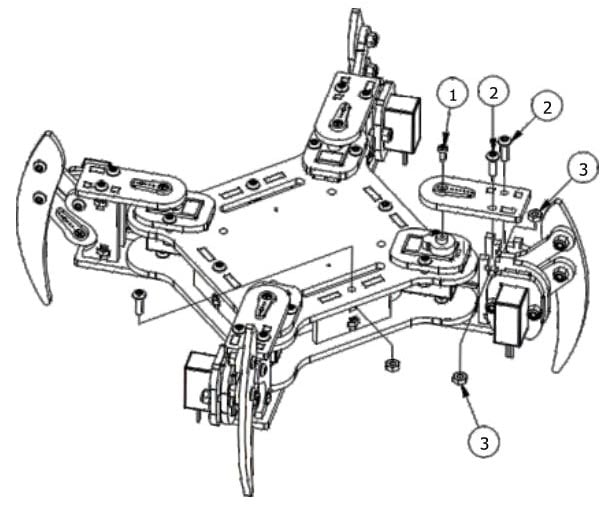After assembly, it will look like this: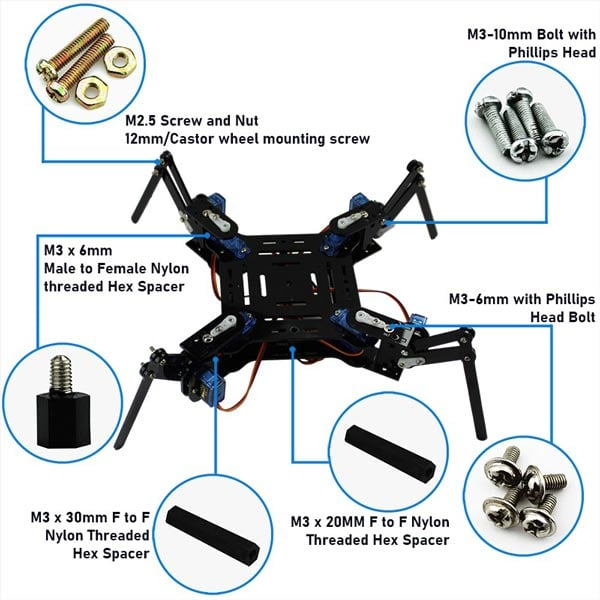### ESP32 Circuit Diagram for Quadruped Spider Robot (8 Servo Motors)

This circuit diagram illustrates the integration of 12 servo motors, enabling locomotion movement in all directions for the robot.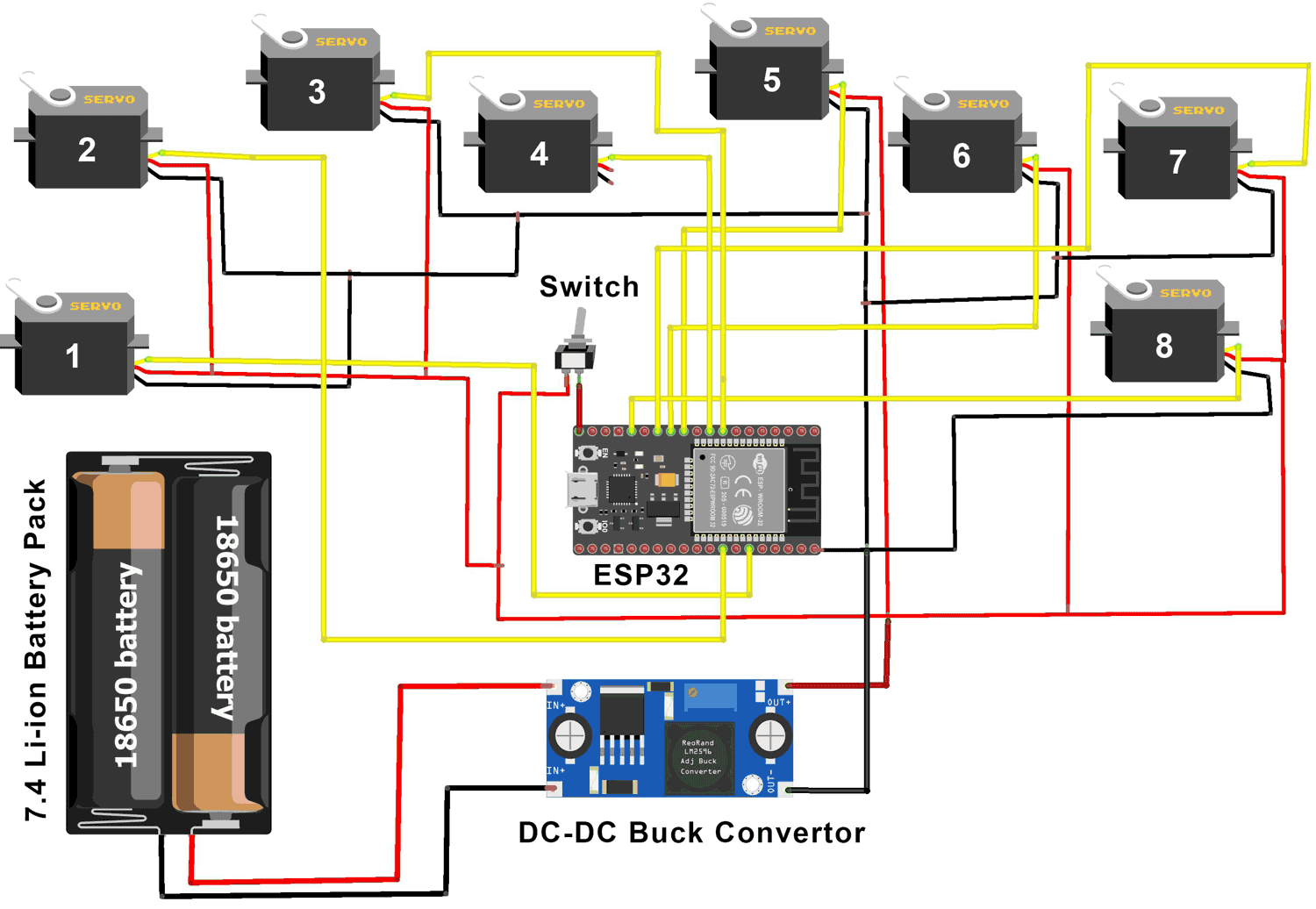The robot consists of four legs, with each leg connected to two servo motors. One servo is responsible for axis rotation (known as the pivot servo), while the other servo controls the lifting motion of the leg (known as the lift servo).

To achieve coordinated movement, the servo motors are connected in a specific sequence or order. The precise order and connections depend on the desired gait pattern and the specific control algorithm being employed. By controlling the movement of these servo motors in a synchronized manner, the robot can achieve stable and coordinated locomotion.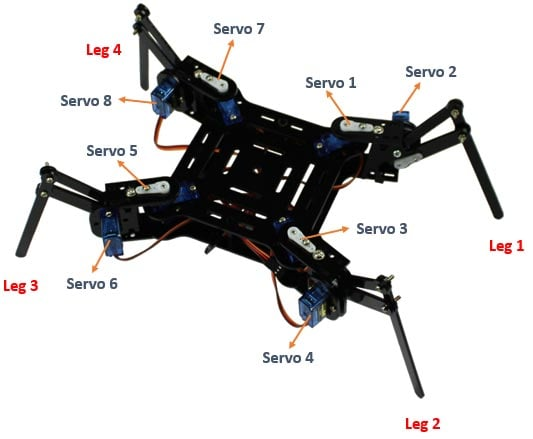ESP32 and Servo Motors:

• Assign PWM (Pulse Width Modulation) capable pins on the ESP32 to control the servos. These pins will generate the necessary PWM signals to adjust the position of each servo motor.
• Connect the signal wire (usually colored yellow or white) from each servo motor to a separate PWM-capable pin on the ESP32.
 Servo1 Pin 21 Servo5 Pin 27 Servo2 Pin 19 Servo6 Pin 14 Servo3 Pin 33 Servo7 Pin 12 Servo4 Pin 25 Servo8 Pin 13

ESP32 and Power supply:

• Connect the power supply's positive terminal to the VCC pins of each servo motor.
• Connect the ground terminal of the power supply to the ground (GND) pins of all servo motors.
• Connect the ground (GND) pin of the ESP32 to the ground terminal (-) of your power supply.
• Connect the Vin pin of the ESP32 to the positive terminal (+) of your power supply with the help of switch.

When working with multiple servo motors in a spider robot, the power requirements exceed what the ESP32 microcontroller can’t provide. Therefore, an external power input is necessary. However, it is crucial to ensure that the input voltage does not exceed 5 volts to prevent damage to the ESP32 microcontroller.

If using a higher voltage battery, use a voltage reducing circuit (DC - DC buck converter)  to lower it to 5 volts. Connect the output of the voltage reducing circuit to both the ESP32 and servo motors. This ensures safe and efficient power supply.

Please note that the specific pin connections and programming code may vary depending on the exact model of the ESP32 board you are using and the servo motors you have. It is important to consult the datasheets and pinout diagrams of your components for accurate pin assignments and voltage requirements. Additionally, ensure you handle the power supply and connections safely, following best practices to avoid any damage to the components.

### ESP32 Quadruped Spider Robot Code Explanation

The spider robot described in this project does not operate through remote control. Instead, it utilizes different functions for various actions such as forward, backward, right, and left movements. These functions are based on the crawl gait (also known as the quadruped gait). The crawl gait pattern is converted into a program and embedded into the ESP32 microcontroller. This program provides instructions to the servo motors, directing them to move the robot's legs into the desired positions for the intended movements. By executing the programmed crawl gait, the spider robot can achieve coordinated and controlled locomotion.

It is important to execute this code #1 before starting the assembly stages. This code is designed to calibrate and adjust the servo motors to their correct angles. By running this code, you can ensure that the servo motors are properly functioning and positioned accurately. This will help prevent any potential issues or complications during the subsequent stages of the assembly process.

Note: Please ensure that you always turn off the switch and disconnect the external power supply before uploading the code to the ESP32. This step is crucial because the switch provides electrical separation between the ESP32 Vin pin and the servos' Vcc pin. This precautionary measure ensures the safety and proper functioning of the components during the code upload process. Remember to follow this procedure each time you upload the code to the ESP32 for the spider robot, maintaining the electrical separation between the ESP32 and the servos to avoid any unwanted complications or damages.

Code #1

```#include <ESP32_Servo.h>    // include servo library
// Define 8 Servos
Servo myServo1; // Front Left Pivot Servo
Servo myServo2; // Front Left Lift Servo
Servo myServo3; // Back Left Pivot Servo
Servo myServo4; // Back Left Lift Servo
Servo myServo5; // Back Right Pivot Servo
Servo myServo6; // Back Right Lift Servo
Servo myServo7; // Front Right Pivot Servo
Servo myServo8; // Front Right Lift Servo
void setup() {
// Attach servos to Arduino Pins
myServo1.attach(21);
myServo2.attach(19);
myServo3.attach(33);
myServo4.attach(25);
myServo5.attach(27);
myServo6.attach(14);
myServo7.attach(12);
myServo8.attach(13);
myServo1.write(90);
myServo2.write(90);
myServo3.write(90);
myServo4.write(90);
myServo5.write(90);
myServo6.write(90);
myServo7.write(90);
myServo8.write(90);
}
void loop() {
}
```

This code #2 includes all the necessary servo motor movements to make your robot functional. Without this code, you will not be able to complete the project successfully. It is crucial for the proper operation of the robot.

Furthermore, the code is well-commented, providing explanations for each block of code. These comments serve as a guide, allowing you to easily identify which block corresponds to specific movements or functionalities. If you need to make any modifications or adjustments, the comments will assist you in understanding the purpose and functionality of each code block.

Code #2

```#include <ESP32_Servo.h>    // include servo library
This line includes the ESP32_Servo library, which provides functions for controlling servo motors using the ESP32 microcontroller.
// Define the delay between function calls (in milliseconds)
const unsigned long FUNCTION_DELAY = 2000; // 2 seconds```

This line declares a global constant named FUNCTION_DELAY, which represents the delay in milliseconds between each function call.

```// Define 8 Servos
Servo myServo1; // Front Left Pivot Servo
Servo myServo2; // Front Left Lift Servo
Servo myServo3; // Back Left Pivot Servo
Servo myServo4; // Back Left Lift Servo
Servo myServo5; // Back Right Pivot Servo
Servo myServo6; // Back Right Lift Servo
Servo myServo7; // Front Right Pivot Servo
Servo myServo8; // Front Right Lift Servo```

This block declares eight Servo objects ‘myServo1’ to ‘myServo8’. Each object represents a servo motor and is associated with a specific function (e.g., pivot or lift) and a specific leg of the spider robot.

```void setup() {
// Attach servos to Arduino Pins
myServo1.attach(21);
myServo2.attach(19);
myServo3.attach(33);
myServo4.attach(25);
myServo5.attach(27);
myServo6.attach(14);
myServo7.attach(12);
myServo8.attach(13);
center_servos();
Serial.println("center");
delay(2000);
}```

Setup function is executed once when the microcontroller starts. It attaches each Servo object to the corresponding Arduino pin using the attach() method. The center_servos() function is called to set all the servo motors to their center position, and a delay of 2 seconds is added for stability.

```void loop() {
// Move Forward 10 step
for (int i = 0; i < 10; i++) {
moveLegServos_Forward();
}
center_servos();
delay(FUNCTION_DELAY);
// Move Backward 10 step
for (int i = 0; i < 10; i++) {
moveLegServos_Backward();
}
center_servos();
delay(FUNCTION_DELAY);
// Move Right 10 step
for (int i = 0; i < 10; i++) {
moveLegServos_Right();
}
center_servos();
delay(FUNCTION_DELAY);
// Move Left 10 step
for (int i = 0; i < 10; i++) {
moveLegServos_Left();
}
center_servos();
delay(FUNCTION_DELAY);
//  dance 10 step
for (int i = 0; i < 10; i++) {
dance();
}
center_servos();
delay(FUNCTION_DELAY);
// pushup 10 step
for (int i = 0; i < 10; i++) {
pushup();
}
center_servos();
delay(FUNCTION_DELAY);
}```

This block contains the ‘loop()’ function, which is executed repeatedly after the ‘setup()’ function. It consists of several ‘for’ loops, each controlling the number of steps for a specific movement. In each iteration, a corresponding movement function is called (‘moveLegServos_Forward()’, ‘moveLegServos_Backward()’,’moveLegServos_Right()’,’moveLegServos_Left()’, ‘pushup()’, ‘dance()’). After each movement, the ‘center_servos()’ function is called to reset all servo motors to their center position. A delay of ‘FUNCTION_DELAY’ milliseconds is introduced between each movement.

```       // Move Spider Robot Forward Function
void moveLegServos_Forward() {
// Control the servo actions for each leg
// Left side leg - Leg 1
moveLeg_Left_Forward(myServo8, myServo7, myServo8);
// Left side leg - Leg 4
moveLeg_Left_Forward(myServo2, myServo1, myServo2);
// Left side leg - Legs 1 and 4
for (int angle = 0; angle <= 90; angle += 2) {
myServo7.write(angle);
myServo1.write(angle);
delay(10);
}
// Right side leg - Leg 2
moveLeg_Right_Forward(myServo6, myServo5, myServo6);
// Right side leg - Leg 3
moveLeg_Right_Forward(myServo4, myServo3, myServo4);
// Right side leg - Legs 2 and 3
for (int angle = 180; angle >= 90; angle -= 2) {
myServo5.write(angle);
myServo3.write(angle);
delay(10);
}
}  ```

This block defines the ‘moveLegServos_Forward()’ function, which controls the servo actions for the spider robot to move forward. It calls the ‘moveLeg_Left_Forward()’ and ‘moveLeg_Right_Forward()’ functions to control the movement of each leg. The function gradually adjusts the servo angles to achieve the desired leg positions for forward movement.

```      // Move Spider Robot Backward Function
void moveLegServos_Backward() {
// Control the servo actions for each leg
// Left side leg - Leg 1
moveLeg_Left_Backward(myServo2, myServo1, myServo2);
// Left side leg - Leg 4
moveLeg_Left_Backward(myServo8, myServo7, myServo8);
// Left side leg - Legs 1 and 4
for (int angle = 180; angle >= 90; angle -= 2) {
myServo1.write(angle);
myServo7.write(angle);
delay(10);
}
// Right side leg - Leg 2
moveLeg_Right_Backward(myServo4, myServo3, myServo4);
// Right side leg - Leg 3
moveLeg_Right_Backward(myServo6, myServo5, myServo6);
// Right side leg - Legs 2 and 3
for (int angle = 0; angle <= 90; angle += 2) {
myServo3.write(angle);
myServo5.write(angle);
delay(10);
}
}```

This block defines the ‘moveLegServos_Backward()’ function, which controls the servo actions for the spider robot to move forward. It calls the ‘moveLeg_Left_Backward()’ and ‘moveLeg_Right_Backward()’ functions to control the movement of each leg. The function gradually adjusts the servo angles to achieve the desired leg positions for Backward movement.

```        // Move Spider Robot Right Function
void moveLegServos_Right() {
// Control the Right turn servo actions for each leg
moveLeg_Right(myServo8, myServo7, myServo1); // Leg 4
moveLeg_Right(myServo6, myServo5, myServo7); // Leg 3
moveLeg_Right(myServo4, myServo3, myServo5); // Leg 2
moveLeg_Right(myServo2, myServo1, myServo3); // Leg 1
}
// Move Spider Robot Left Function
void moveLegServos_Left() {
// Control the Left turn servo actions for each leg
moveLeg_Left(myServo8, myServo7, myServo1); // Leg 4
moveLeg_Left(myServo6, myServo5, myServo7); // Leg 3
moveLeg_Left(myServo4, myServo3, myServo5); // Leg 2
moveLeg_Left(myServo2, myServo1, myServo3); // Leg 1
}```

In this block of  code, the ‘moveLegServos_Right()’ and ‘moveLegServos_Left()’ functions control the servo actions for the spider robot to move right and left, respectively.

These functions coordinate the movements of the leg servos to achieve the desired motion of the spider robot, either to the right or left. Each function calls the appropriate ‘moveLeg_Right()’ or ‘moveLeg_Left()’ function for each leg of the robot, passing the corresponding servo objects as arguments.

```       //  Spider Robot dance Function
void dance(){
// Move the selected servos from 0 to 180 degrees
for (int angle = 0; angle <= 180; angle += 2) {
myServo1.write(angle);
myServo3.write(angle);
myServo5.write(angle);
myServo7.write(angle);
delay(10);  // Delay between each angle change (adjust as needed)
}
// Move the selected servos from 180 to 0 degrees
for (int angle = 180; angle >= 0; angle -= 2) {
myServo1.write(angle);
myServo3.write(angle);
myServo5.write(angle);
myServo7.write(angle);
delay(10);  // Delay between each angle change (adjust as needed)
}
}
//  Spider Robot pushup Function
void pushup(){
// Move the selected servos from 0 to 180 degrees
for (int angle = 0; angle <= 180; angle += 2) {
myServo2.write(angle);
myServo4.write(angle);
myServo6.write(angle);
myServo8.write(angle);
delay(10);  // Delay between each angle change (adjust as needed)
}
// Move the selected servos from 180 to 0 degrees
for (int angle = 180; angle >= 0; angle -= 2) {
myServo2.write(angle);
myServo4.write(angle);
myServo6.write(angle);
myServo8.write(angle);
delay(10);  // Delay between each angle change (adjust as needed)
}
}```

This block defines the pushup() and  dance() function, which controls the servo actions for the spider robot to perform a pushup and dance motion. It alternates the servo angles between 0 and 180 degrees for the selected servos (myServo1, myServo3, myServo5, myServo7) to achieve the dance motion effect and (myServo2, myServo4, myServo6, myServo8) for pushup motion effect.

```          // Move Spider Robot Forward logic
void moveLeg_Left_Forward(Servo& liftServo, Servo& pivotServo , Servo& oppositePivotServo) {
// Move the lift servo from 90 to 180
for (int angle = 90; angle <= 180; angle += 2) {
liftServo.write(angle);
delay(10);
}
// Move the pivot servo from 90 to 0
for (int angle = 90; angle >= 0; angle -= 2) {
pivotServo.write(angle);
delay(10);
}
// Move the lift servo from 180 to 90
for (int angle = 180; angle >= 90; angle -= 2) {
liftServo.write(angle);
delay(10);
}
}
void moveLeg_Right_Forward(Servo& liftServo, Servo& pivotServo , Servo& oppositePivotServo) {
// Move the lift servo from 90 to 180
for (int angle = 90; angle <= 180; angle += 2) {
liftServo.write(angle);
delay(10);
}
// Move the pivot servo from 90 to 180
for (int angle = 90; angle <= 180; angle += 2) {
pivotServo.write(angle);
delay(10);
}
// Move the lift servo from 180 to 90
for (int angle = 180; angle >= 90; angle -= 2) {
liftServo.write(angle);
delay(10);
}
}
// Move Spider  Robot Backward logic
void moveLeg_Left_Backward(Servo& liftServo, Servo& pivotServo, Servo& oppositePivotServo) {
// Move the lift servo from 90 to 180
for (int angle = 90; angle <= 180; angle += 2) {
liftServo.write(angle);
delay(10);
}
// Move the pivot servo from 90 to 180
for (int angle = 90; angle <= 180; angle += 2) {
pivotServo.write(angle);
delay(10);
}
// Move the lift servo from 180 to 90
for (int angle = 180; angle >= 90; angle -= 2) {
liftServo.write(angle);
delay(10);
}
}
void moveLeg_Right_Backward(Servo& liftServo, Servo& pivotServo, Servo& oppositePivotServo) {
// Move the lift servo from 90 to 180
for (int angle = 90; angle <= 180; angle += 2) {
liftServo.write(angle);
delay(10);
}
// Move the pivot servo from 90 to 0
for (int angle = 90; angle >= 0; angle -= 2) {
pivotServo.write(angle);
delay(10);
}
// Move the lift servo from 180 to 90
for (int angle = 180; angle >= 90; angle -= 2) {
liftServo.write(angle);
delay(10);
}
}```

This block of code includes four functions ‘moveLeg_Left_Forward()’, ‘moveLeg_Right_Forward()’, ‘moveLeg_Left_Backward()’, and ‘moveLeg_Right_Backward()’.

moveLeg_Left_Forward(): This function is responsible for moving the left leg of the spider robot forward. It takes three servo objects as parameters: liftServo, pivotServo, and oppositePivotServo. The function first moves the liftServo from 90 to 180 degrees in increments of 2 degrees. Then, it moves the pivotServo from 90 to 0 degrees in the same increment. Finally, it moves the liftServo back from 180 to 90 degrees. Each movement is accompanied by a delay of 10 milliseconds using the delay(10) statement.

moveLeg_Right_Forward(): This function is responsible for moving the right leg of the spider robot forward. It has a similar structure to moveLeg_Left_Forward(), but with the addition of an extra movement. After moving the liftServo and pivotServo as described above, it moves the oppositePivotServo from 0 to 90 degrees. The purpose of this additional movement is to coordinate the leg motions for forward movement.

moveLeg_Left_Backward(): This function is responsible for moving the left leg of the spider robot backward. It follows a similar structure to moveLeg_Left_Forward(), but with some changes in the angle increments and directions. The pivotServo is moved from 90 to 180 degrees, and the liftServo from 180 to 90 degrees. These changes result in the leg moving in the opposite direction.

moveLeg_Right_Backward(): This function is responsible for moving the right leg of the spider robot backward. It has a similar structure to moveLeg_Left_Backward(), with the addition of an extra movement. After moving the liftServo and pivotServo as described above, it moves the oppositePivotServo from 90 to 0 degrees.

```             // Move Spider  Robot Right logic
void moveLeg_Right(Servo& liftServo, Servo& pivotServo , Servo& oppositePivotServo) {
// Move the lift servo from 90 to 180
for (int angle = 90; angle <= 180; angle += 2) {
liftServo.write(angle);
delay(10);
}
// Move the pivot servo from 90 to 0
for (int angle = 90; angle >= 0; angle -= 2) {
pivotServo.write(angle);
delay(10);
}
// Move the opposite pivot servo from 0 to 90
for (int angle = 0; angle <= 90; angle += 2) {
oppositePivotServo.write(angle);
delay(10);
}
// Move the lift servo from 90 to 180
for (int angle = 180; angle >= 90; angle -= 2) {
liftServo.write(angle);
delay(10);
}
}
// Move Spider  Robot Left logic
void moveLeg_Left(Servo& liftServo, Servo& pivotServo , Servo& oppositePivotServo) {
// Move the lift servo from 90 to 180
for (int angle = 90; angle <= 180; angle += 2) {
liftServo.write(angle);
delay(10);
}
// Move the pivot servo from 90 to 180
for (int angle = 90; angle <= 180; angle += 2) {
pivotServo.write(angle);
delay(10);
}
// Move the opposite pivot servo from 180 to 90
for (int angle = 180; angle >= 90; angle -= 2) {
oppositePivotServo.write(angle);
delay(10);
}
// Move the lift servo from 180 to 90
for (int angle = 180; angle >= 90; angle -= 2) {
liftServo.write(angle);
delay(10);
}
}```

This block of  code includes two functions moveLeg_Right() and moveLeg_Left(). Here's an explanation of each function:

moveLeg_Right(): This function is responsible for moving the right leg of the spider robot in a right direction or turn. It takes three servo objects as parameters: liftServo, pivotServo, and oppositePivotServo. The function starts by moving the liftServo from 90 to 180 degrees in increments of 2 degrees. Then, it moves the pivotServo from 90 to 0 degrees in the same increment. Next, it moves the oppositePivotServo from 0 to 90 degrees. Finally, it moves the liftServo back from 180 to 90 degrees. Each movement is accompanied by a delay of 10 milliseconds using the delay(10) statement.

moveLeg_Left(): This function is responsible for moving the left leg of the spider robot in a left direction or turn. It follows a similar structure to moveLeg_Right(), but with some changes in the angle increments and directions. The pivotServo is moved from 90 to 180 degrees, and the oppositePivotServo from 180 to 90 degrees. These changes result in the leg moving in the opposite direction.

```      // All Servos Centor function
void center_servos() {
myServo1.write(90);
myServo2.write(90);
myServo3.write(90);
myServo4.write(90);
myServo5.write(90);
myServo6.write(90);
myServo7.write(90);
myServo8.write(90);
}```

This block defines the center_servos() function, which sets all servo motors to their center position by writing an angle of 90 degrees to each servo.

Overall, the code provides a basic framework for controlling the spider robot using the ESP32 microcontroller and servo motors, enabling it to perform various movements and actions.

Code

Code #1

```#include <ESP32_Servo.h>    // include servo library
// Define 8 Servos
Servo myServo1; // Front Left Pivot Servo
Servo myServo2; // Front Left Lift Servo
Servo myServo3; // Back Left Pivot Servo
Servo myServo4; // Back Left Lift Servo
Servo myServo5; // Back Right Pivot Servo
Servo myServo6; // Back Right Lift Servo
Servo myServo7; // Front Right Pivot Servo
Servo myServo8; // Front Right Lift Servo

void setup()
{

// Attach servos to Arduino Pins
myServo1.attach(21);
myServo2.attach(19);
myServo3.attach(33);
myServo4.attach(25);
myServo5.attach(27);
myServo6.attach(14);
myServo7.attach(12);
myServo8.attach(13);

myServo1.write(90);
myServo2.write(90);
myServo3.write(90);
myServo4.write(90);
myServo5.write(90);
myServo6.write(90);
myServo7.write(90);
myServo8.write(90);
}
void loop()
{
}```

Code #2

```#include <ESP32_Servo.h>    // include servo library

// Define the delay between function calls (in milliseconds)
const unsigned long FUNCTION_DELAY = 2000; // 2 seconds

const unsigned long t = 2;
const unsigned long tt = 5;

// Define 8 Servos
Servo myServo1; // Front Left Pivot Servo
Servo myServo2; // Front Left Lift Servo
Servo myServo3; // Back Left Pivot Servo
Servo myServo4; // Back Left Lift Servo
Servo myServo5; // Back Right Pivot Servo
Servo myServo6; // Back Right Lift Servo
Servo myServo7; // Front Right Pivot Servo
Servo myServo8; // Front Right Lift Servo

void setup()
{

// Attach servos to Arduino Pins
myServo1.attach(21);
myServo2.attach(19);
myServo3.attach(33);
myServo4.attach(25);
myServo5.attach(27);
myServo6.attach(14);
myServo7.attach(12);
myServo8.attach(13);

center_servos();
Serial.println("center");
delay(2000);
}

void loop()
{
//Move Forward 10 step
for (int i = 0; i < 10; i++) {
moveLegServos_Forward();
}
center_servos();
delay(FUNCTION_DELAY);

// Move Backward 10 step
for (int i = 0; i < 10; i++) {
moveLegServos_Backward();
}
center_servos();
delay(FUNCTION_DELAY);
// Move Right 10 step
for (int i = 0; i < 10; i++) {
moveLegServos_Right();
}
center_servos();
delay(FUNCTION_DELAY);
// Move Left 10 step
for (int i = 0; i < 10; i++) {
moveLegServos_Left();
}
center_servos();
delay(FUNCTION_DELAY);
//  dance 10 step
for (int i = 0; i < 5; i++) {
dance();
}
center_servos();
delay(FUNCTION_DELAY);
// pushup 10 step
for (int i = 0; i < 10; i++) {
pushup();
}
center_servos();
delay(FUNCTION_DELAY);
}

// Move Spider Robot Forward Function
void moveLegServos_Forward()
{

// Control the servo actions for each leg

// Left side leg - Leg 1
moveLeg_Left_Forward(myServo8, myServo7, myServo8);

// Left side leg - Leg 4
moveLeg_Left_Forward(myServo2, myServo1, myServo2);

// Left side leg - Legs 1 and 4
for (int angle = 0; angle <= 90; angle += 2) {
myServo7.write(angle);
myServo1.write(angle);
delay(tt);
}

// Right side leg - Leg 2
moveLeg_Right_Forward(myServo6, myServo5, myServo6);

// Right side leg - Leg 3
moveLeg_Right_Forward(myServo4, myServo3, myServo4);

// Right side leg - Legs 2 and 3
for (int angle = 180; angle >= 90; angle -= 2) {
myServo5.write(angle);
myServo3.write(angle);
delay(tt);
}
}

// Move Spider Robot Backward Function
void moveLegServos_Backward()
{

// Control the servo actions for each leg
// Left side leg - Leg 1
moveLeg_Left_Backward(myServo2, myServo1, myServo2);

// Left side leg - Leg 4
moveLeg_Left_Backward(myServo8, myServo7, myServo8);

// Left side leg - Legs 1 and 4
for (int angle = 180; angle >= 90; angle -= 2) {
myServo1.write(angle);
myServo7.write(angle);
delay(tt);
}

// Right side leg - Leg 2
moveLeg_Right_Backward(myServo4, myServo3, myServo4);

// Right side leg - Leg 3
moveLeg_Right_Backward(myServo6, myServo5, myServo6);

// Right side leg - Legs 2 and 3
for (int angle = 0; angle <= 90; angle += 2) {
myServo3.write(angle);
myServo5.write(angle);
delay(tt);
}
}

// Move Spider Robot Right Function
void moveLegServos_Right() {
// Control the Right turn servo actions for each leg
moveLeg_Right(myServo8, myServo7, myServo5); // Leg 4
moveLeg_Right(myServo2, myServo1, myServo7); // Leg 3
moveLeg_Right(myServo4, myServo3, myServo1); // Leg 2
moveLeg_Right(myServo6, myServo5, myServo3); // Leg 1
}

// Move Spider Robot Left Function
void moveLegServos_Left() {
// Control the Left turn servo actions for each leg
moveLeg_Left(myServo8, myServo7, myServo1); // Leg 4
moveLeg_Left(myServo6, myServo5, myServo7); // Leg 3
moveLeg_Left(myServo4, myServo3, myServo5); // Leg 2
moveLeg_Left(myServo2, myServo1, myServo3); // Leg 1
}

//  Spider Robot dance Function
void dance(){
// Move the selected servos from 0 to 180 degrees
for (int angle = 0; angle <= 180; angle += 2) {
myServo1.write(angle);
myServo3.write(angle);
myServo5.write(angle);
myServo7.write(angle);
delay(t);  // Delay between each angle change (adjust as needed)
}

// Move the selected servos from 180 to 0 degrees
for (int angle = 180; angle >= 0; angle -= 2) {
myServo1.write(angle);
myServo3.write(angle);
myServo5.write(angle);
myServo7.write(angle);
delay(t);  // Delay between each angle change (adjust as needed)
}
center_servos();
delay(100);
lean_left();
delay(300);
lean_right();
delay(300);
lean_left();
delay(300);
lean_right();
delay(300);
lean_left();
delay(300);
lean_right();
delay(300);
lean_left();
delay(300);
lean_right();
delay(500);
center_servos();
delay(300);
bow();
center_servos();
}

//  Spider Robot pushup Function
void pushup(){
// Move the selected servos from 0 to 180 degrees
for (int angle = 0; angle <= 180; angle += 2) {
myServo2.write(angle);
myServo4.write(angle);
myServo6.write(angle);
myServo8.write(angle);
delay(10);  // Delay between each angle change (adjust as needed)
}

// Move the selected servos from 180 to 0 degrees
for (int angle = 180; angle >= 0; angle -= 2) {
myServo2.write(angle);
myServo4.write(angle);
myServo6.write(angle);
myServo8.write(angle);
delay(10);  // Delay between each angle change (adjust as needed)
}
}

// Move Spider Robot Forward logic

void moveLeg_Left_Forward(Servo& liftServo, Servo& pivotServo , Servo& oppositePivotServo)
{
// Move the lift servo from 90 to 180
for (int angle = 90; angle <= 180; angle += 2) {
liftServo.write(angle);
delay(tt);
}

// Move the pivot servo from 90 to 0
for (int angle = 90; angle >= 0; angle -= 2) {
pivotServo.write(angle);
delay(tt);
}

// Move the lift servo from 180 to 90
for (int angle = 180; angle >= 90; angle -= 2) {
liftServo.write(angle);
delay(tt);
}
}
void moveLeg_Right_Forward(Servo& liftServo, Servo& pivotServo , Servo& oppositePivotServo)
{
// Move the lift servo from 90 to 180
for (int angle = 90; angle <= 180; angle += 2) {
liftServo.write(angle);
delay(tt);
}

// Move the pivot servo from 90 to 180
for (int angle = 90; angle <= 180; angle += 2) {
pivotServo.write(angle);
delay(tt);
}

// Move the lift servo from 180 to 90
for (int angle = 180; angle >= 90; angle -= 2) {
liftServo.write(angle);
delay(tt);
}
}
// Move Spider  Robot Backward logic
void moveLeg_Left_Backward(Servo& liftServo, Servo& pivotServo, Servo& oppositePivotServo)
{
// Move the lift servo from 90 to 180
for (int angle = 90; angle <= 180; angle += 2) {
liftServo.write(angle);
delay(tt);
}

// Move the pivot servo from 90 to 180
for (int angle = 90; angle <= 180; angle += 2) {
pivotServo.write(angle);
delay(tt);
}

// Move the lift servo from 180 to 90
for (int angle = 180; angle >= 90; angle -= 2) {
liftServo.write(angle);
delay(tt);
}
}

void moveLeg_Right_Backward(Servo& liftServo, Servo& pivotServo, Servo& oppositePivotServo)
{
// Move the lift servo from 90 to 180
for (int angle = 90; angle <= 180; angle += 2) {
liftServo.write(angle);
delay(tt);
}

// Move the pivot servo from 90 to 0
for (int angle = 90; angle >= 0; angle -= 2) {
pivotServo.write(angle);
delay(tt);
}

// Move the lift servo from 180 to 90
for (int angle = 180; angle >= 90; angle -= 2) {
liftServo.write(angle);
delay(tt);
}
}

// Move Spider  Robot Right logic
void moveLeg_Right(Servo& liftServo, Servo& pivotServo , Servo& oppositePivotServo)
{
// Move the lift servo from 90 to 180
for (int angle = 90; angle <= 180; angle += 2) {
liftServo.write(angle);
delay(t);
}

// Move the pivot servo from 90 to 0
for (int angle = 90; angle >= 0; angle -= 2) {
pivotServo.write(angle);
delay(t);
}

// Move the opposite pivot servo from 0 to 90
for (int angle = 0; angle <= 90; angle += 2) {
oppositePivotServo.write(angle);
delay(t);
}

// Move the lift servo from 90 to 180
for (int angle = 180; angle >= 90; angle -= 2) {
liftServo.write(angle);
delay(t);
}
}

// Move Spider  Robot Left logic
void moveLeg_Left(Servo& liftServo, Servo& pivotServo , Servo& oppositePivotServo)
{
// Move the lift servo from 90 to 180
for (int angle = 90; angle <= 180; angle += 2) {
liftServo.write(angle);
delay(t);
}

// Move the pivot servo from 90 to 180
for (int angle = 90; angle <= 180; angle += 2) {
pivotServo.write(angle);
delay(t);
}

// Move the opposite pivot servo from 180 to 90
for (int angle = 180; angle >= 90; angle -= 2) {
oppositePivotServo.write(angle);
delay(t);
}

// Move the lift servo from 180 to 90
for (int angle = 180; angle >= 90; angle -= 2) {
liftServo.write(angle);
delay(t);
}
}

void bow()
{
center_servos();
delay(200);
myServo2.write(15);
myServo8.write(15);
delay(700);
myServo2.write(90);
myServo8.write(90);
delay(700);
}

void lean_left()
{
myServo2.write(15);
myServo4.write(15);
myServo6.write(150);
myServo8.write(150);
}

void lean_right()
{
myServo2.write(150);
myServo4.write(150);
myServo6.write(15);
myServo8.write(15);
}

// All Servos Centor function
void center_servos()
{
myServo1.write(90);
myServo2.write(90);
myServo3.write(90);
myServo4.write(90);
myServo5.write(90);
myServo6.write(90);
myServo7.write(90);
myServo8.write(90);
}
```
Tags